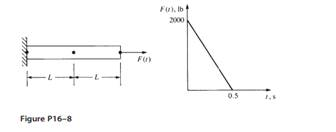### Create an Account

Home / Questions / For the bar shown in Figure P16 8 determine the nodal displacements velocities and acceler...

# For the bar shown in Figure P16 8 determine the nodal displacements velocities and accelerations for five time steps using two finite elements For simplicity of calculations

For the bar shown in Figure P16–8, determine the nodal displacements, velocities, and accelerations for five time steps using two finite elements. For simplicity of calculations, let E = 1 × 106 psi, ρ = 1 lb-s2/in4, A = 1 in2, and L = 100 in. Use Newmark’s method and Wilson’s method.Aug 01 2020 View more View LessSubscribe To Get Solution